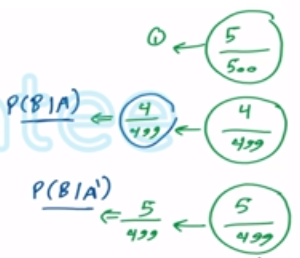Need Help?

Subscribe to Probability

###### \${selected_topic_name}
• Notes

$\text { If } P(A | B)=0.3, P(B)=0.8, \text { and } P(A)=0.3, \text { are }$
the events $B$ and the complement of $A$ independent?

$P(A | B)=0.3$
$P(B)=0.8$
$P(A)=0.3$

$B, A^\prime$ independed??

$P\left(A^{\prime}\right)=1-P(A)=0.7$

$P\left(A^{\prime} / B\right)=1-P(A | B)$

$=1-0.3=0.7$

$P\left(A^{\prime} | B\right)=P\left(A^{\prime}\right) \Rightarrow A^{\prime}, B \rightarrow$ independent

A batch of 500 containers for frozen orange juice con-
tains 5 that are defective. Two are selected, at random, without
replacement, from the batch. Let $A$ and $B$ denote the events
that the first and second container selected is defective, re-
spectively.

(a) Are $A$ and $B$ independent events?
(b) If the sampling were done with replacement, would $A$ and
$B$ be independent?

batch $\rightarrow 500$

5 defective

495

$A \rightarrow$ first is defective
$B \rightarrow$ second is defective

a) $P(A | B)=P(A)$
$P(B | A)=P(B)$$P(A)=\frac{5}{500}=\frac{1}{100}$

$P(B)=P(B | A) P(A)+P(B | A^{\prime}) P(A^{\prime})$

$=\frac{4}{499} \times \frac{5}{500}+\frac{5}{499} \times \frac{495}{500}=\frac{5}{500}=\frac{1}{100}$

$P(B | A) \neq P(B)$

$\text { Not independet }$

b) $\rightarrow A, B \rightarrow$ independet